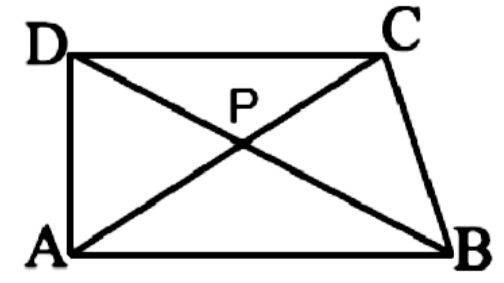Home/Class 7/Maths/

$$ABCD$$ is quadrilateral. Is $$AB + BC + CD + DA < 2 (AC + BD)$$Speed
00:00
04:07## QuestionMathsClass 7

$$ABCD$$ is quadrilateral. Is $$AB + BC + CD + DA < 2 (AC + BD)$$

Yes
4.64.6## Solution

Let us consider $$ABCD$$ is quadrilateral and $$P$$ is the point where the diagonals are intersect. As shown in the figure below.We know that,
The sum of the length of any two sides is always greater than the third side.
Now consider the $$ΔPAB$$,
Here, $$PA+PB>AB$$ … [equation i]
Then, consider the $$ΔPBC$$
Here, $$PB+PC>BC$$ … [equation ii]
Consider the $$ΔPCD$$
Here, $$PC+PD>CD$$ … [equation iii]
Consider the $$ΔPDA$$
Here, $$PD+PA>DA$$ … [equation iv]
By adding equation [i], [ii], [iii] and [iv], we get,$$PA+PB+PB+PC+PC+PD+PD+PA>AB+BC+CD+DA$$
$$\implies 2PA+2PB+2PC+2PD>AB+BC+CD+DA$$
$$\implies 2PA+2PC+2PB+2PD>AB+BC+CD+DA$$
$$\implies 2(PA+PC)+2(PB+PD)>AB+BC+CD+DA$$
From the figure we have, $$AC = PA + PC$$ and $$BD = PB + PD$$
Then,
$$2AC+2BD>AB+BC+CD+DA$$
$$\implies 2(AC+BD)>AB+BC+CD+DA$$
Hence, the given expression is true.